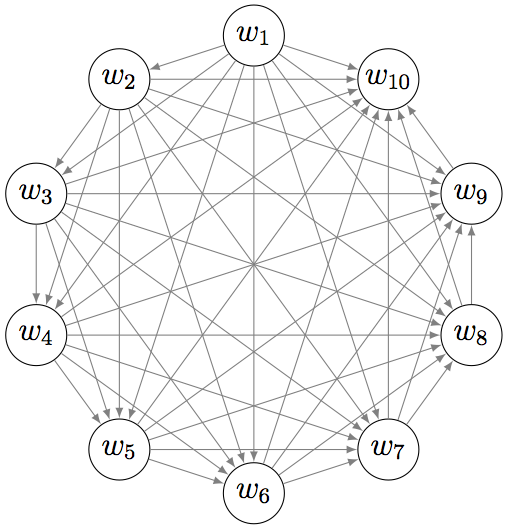# Topic Coherence To Evaluate Topic Models

Human judgment not being correlated to perplexity (or likelihood of unseen documents) is the motivation for more work trying to model the human judgment. This is by itself a hard task as human judgment is not clearly defined; for example, two experts can disagree on the usefulness of a topic.

One can classify the methods addressing this problem into two categories. \textit{Intrinsic} methods that do not use any external source or task from the dataset, whereas \textit{extrinsic} methods use the discovered topics for external tasks, such as information retrieval [Wei06], or use external statistics to evaluate topics.

As an early intrinsic method, [Alsumait09] define measures based on three prototypes of junk and insignificant topics. The three prototypes for junk topics are the uniform word-distribution, the empirical corpus word-distribution, and the uniform document-distribution: $$p(w | \text{topic}) \propto 1 \qquad p(w | \text{topic}) \propto \text{count}(w \text{ in corpus}) \qquad p(d | \text{topic}) \propto 1$$ Then a topic significance score is computed from various dissimilarities and similarities (KL divergence, cosine, and correlation) to these three prototypes. However, the significance score is a complicated function with free parameters, that seem to be arbitrarily chosen, so the risk of overfitting the two datasets used for experiments is high.

### Topic coherence

The state-of-the-art in terms of topic coherence are the intrinsic measure UMass and the extrinsic measure UCI, both based on the same high level idea. Both measure compute the sum $$\text{Coherence} = \sum_{i \lt j} \text{score}(w_i, w_j)$$ of pairwise scores on the words $w_1$, ..., $w_n$ used to describe the topic, usually the top $n$ words by frequency $p(w|k)$. This measure can be seen as the sum of all edges on complete graph.Both topic coherence measures UCI and UMass are based on the sum $\sum_{i \lt j} \text{score}(w_i, w_j)$ of the pairwise scores of the $n$ top words $w_1$, ..., $w_n$ of the topic.

Notation. Let's define $D(w_i)$ as the count of documents containing the word $w_i$, $D(w_i, w_j)$ the count of documents containing both words $w_i$ and $w_j$, and $D$ the total number or documents in the corpus. The corpus used to compute the counts is specified in a subscript of symbol $D$. For example, $D_{\text{Wikipedia}}(w_i)$ it the count of documents of the Wikipedia corpus containing the word $w_i$. When no subscript is specified, the corpus used is the corpus on which the model have been trained.

### Extrinsic UCI measure

The UCI measure introduced by [Newman10a] uses as pairwise score function the Pointwise Mutual Information (PMI) $$\text{score}_{\text{UCI}}(w_i, w_j) = \log \frac{p(w_i, w_j)}{p(w_i)p(w_j)}$$ where $p(w)$ represents the probability of seeing $w_i$ in a random document, and $p(w_i, w_j)$ the probability of seeing both $w_i$ and $w_j$ co-occurring in a random document. Those probabilities are empirically estimated from an external dataset such as Wikipedia: $$p(w_i) = \frac{D_{\text{Wikipedia}}(w_i)}{D_{\text{Wikipedia}}} \qquad \text{and} \qquad p(w_i, w_j) = \frac{D_{\text{Wikipedia}}(w_i, w_j)}{D_{\text{Wikipedia}}}.$$

Given the score function, we are free to choose the corpus to compute the empirical probabilities. [Newman10a] chose three external corpus to evaluate them (Wikipedia, Google 2-grams, and Medline) but not the corpus that generated the topics. The argument given is that using the same dataset would reinforce noise or unusual word statistics. However, some intrinsic topic coherence measures have been developed since, that are also better correlated to human judgment than perplexity Mimno11a. It may be worth comparing intrinsic and extrinsic PMI-based measures.

### Intrinsic UMass measure

The UMass measure introduced by [Mimno11a] uses as pairwise score function $$\text{score}_{\text{UMass}}(w_i, w_j) = \log \frac{D(w_i, w_j) + 1}{D(w_i)}$$ which is the empirical conditional log-probability $\log p(w_j|w_i) = \log \frac{p(w_i, w_j)}{p(w_j)}$ smoothed by adding one to $D(w_i, w_j)$.

The score function is not symmetric as it is an increasing function of the empirical probability $p(w_j|w_i)$, where $w_i$ is more common than $w_j$, words being ordered by decreasing frequency $p(w|k)$. So this score measures how much, within the words used to describe a topic, a common word is in average a good predictor for a less common word.

As the pairwise score used by the UMass measure is not symmetric, the order of the arguments matters. UMass measure is computing $p(\text{rare word } | \text{ common word})$, how much a common word triggers a rarer word. However, in human word association, high frequency words are more likely to be used as response words than low frequency words [Steyvers06]. It would be interesting to understand the effect of this choice by doing more experiments and comparing the two options.Quentin Pleplé
May 2013